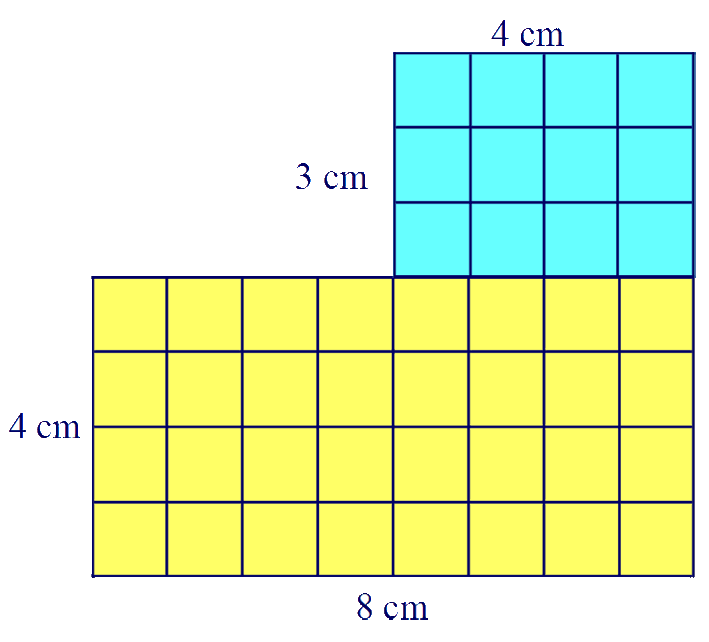# Shape and Space - Area of 2D Shapes

## The Area of a 2D shape is the space within the perimeter. It is the amount of surface that the 2D shape covers.

### The units of area are the square of the unit used to measure the shape. If the unit used is centimetres then the unit of measurement is square centimetres, cm². If metres are used then the unit of measurement is square metres, m². For larger areas, like countries we would use square kilometres, km².

This idea of square units can be difficult to understand - I explain it as the number of squares of that size, 1 cm, that you would need to cover the shape. Lets take an example of a rectangle that is 4 cm wide and 3 cm high. How many squares that are 1cm wide and 1 cm high will fit in the shape? The best way is to draw it or even cut out the 1cm squares and make the shape.You can clearly see that it takes twelve, one centimetre squares to cover the shape -
so we can say that the area of the shape is twelve centimetre squares - we write this as, 12 cm² .
We say this as "twelve square centimetres" or "twelve centimetres squared".

Clearly we cant do this for every shape that we wish to find the area of. For a rectangle, all we need to do is count how many squares would fit across the rectangle, in this case 4. Then count how many squares would fit up the rectangle, in this case 3. Then multiply them together - 4 x 3 = 12 cm².

For more complex shapes, you may have to split the shape into two or more rectangle and work out the area of each one individually and then combine the results.This shape has been split into two rectangles.

The blue rectangle has an area of 4 x 3 = 12 cm²

The yellow rectangle has an area of 8 x 4 = 32 cm²

Add the results .... 32 + 12 = 44 cm²

So the area of the total shape is 44 cm²

Your child may also be asked to find the area of triangles. This may initially look to be more complicated until you understand that a triangle is half of a rectangle.The area of the rectangle is width x height, 8 x 4 = 32 cm²

If you count up the amount of blue squares which form the triangle, you should get 12 whole blue squares and 8 half blue squares (equivalent to a further 4 whole blue squares),
so the area of the blue triangle is 12 + 4 = 16 cm²

Therefore the area of the triangle is half the area of the rectangle. So we can say that the area of the triangle is half the base multiplied by the height. ½ x 8 x 4 = 16 cm²

Practice working with Perimeter and Area with these worksheets from Primary Resources